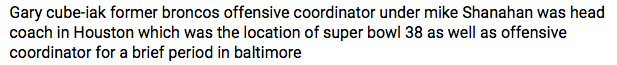## Wednesday, December 30, 2015

### 66 84 174 | Gary Kubiak, Denver Broncos Coach, San Francisco Ties (Carolina Panthers too)

Gary = 7+1+9+7 = 24
Wayne = 5+1+7+5+5 = 23
Kubiak = 2+3+2+9+1+2 = 19
Gary Wayne Kubiak = 66 (NFL = 66, Jewish Gematria) (Season of First Super Bowl, '66)
Gary Kubiak = 43

Gary = 7+1+18+25 = 51
Wayne = 23+1+25+14+5 = 68
Kubiak = 11+21+2+9+1+11 = 55
Gary Wayne Kubiak = 174 (Carolina Panthers = 174)
Gary Kubiak = 106

8/15/1961 = 8+15+19+61 = 103
8/15/1961 = 8+15+(1+9+6+1) = 40
8/15/1961 = 8+1+5+1+9+6+1 = 31
8/15/61 = 8+15+61 = 84 (United States of America)

It is interesting to note that Kubiak has ties as a player to the Denver Broncos, and as a coach to the San Francisco 49ers.  Again, the 49ers are hosting Super Bowl 50, and the Denver Broncos have a strong connection to Levi's Stadium, where the 49ers now play.  Kubiak also has connections to the Ravens, who the 49ers lost to in Super Bowl 47, the one with the '33 minute blackout'.What a reader thinks.What a reader submitted.

1.1.